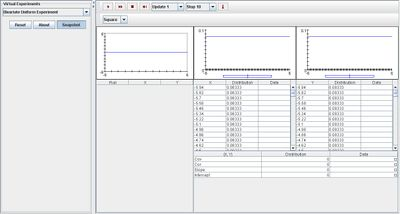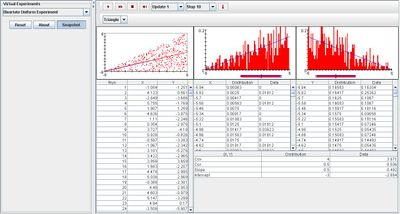# SOCR EduMaterials Activities BivariteUniformExperiment

(diff) ← Older revision | Latest revision (diff) | Newer revision → (diff)

## Description

The experiment generates a random point (X,Y) from a uniform distribution on a region of the plane. From the following regions, any region can be selected with the list box directly above the graphs on the left: the square -6 < x < 6, -6 < y < 6; the triangle -6 < y < x < 6; and the circle of radius 6 centered at the origin. In the scatter plot located to the left shows a red dot which represents the generated random point, and the distribution regression line and sample regression line is also displayed in blue and red, respectively. The second and third graphs illustrate the distribution and sample density and moments of X and Y. The first data table numerically records the coordinates of each random point. In the second and third tables, the distribution and sample moments are recorded. Located below is a table displaying the distribution, covariance, correlation slope, and intercept are recoded.

## Goal

To offer a simple simulation for a vector variable in a finite positive area of a square, triangle, or circle to be randomly placed and obtaining its information (e.g. marginal distributions, coordinates, conditional density, etc.)

## Experiment

Go to the SOCR Experiments and select the Bivariate Uniform Experiment from the drop-down list of experiments on the top left. The image below shows the initial view of this experiment:When pressing the play button, one trial will be executed and recorded in the distribution table below. The fast forward button symbolizes the nth number of trials to be executed each time. The stop button ceases any activity and is helpful when the experimenter chooses “continuous,” indicating an infinite number of events. The fourth button will reset the entire experiment, deleting all previous information and data collected. The “update” scroll indicates nth number of trials (1, 10, 100, or 1000) performed when selecting the fast forward button and the “stop” scroll indicates the maximum number of trials in the experiment.

As the number of runs increase, the displayed graphs of the collected points take shape of the chosen region of area. The image below displays the experiment for the triangle region after many trials.## Applications

This SOCR Experiment is an accessible method that explores the treatment of a large variety of types of dependence. It is useful for statistical models where the random points influence the overall outcome of the event.

Suppose researchers want to illustrate the proportion of students who graduate high school in the United States. By using this java applet, a uniform horizontal density graph may be displayed to demonstrate a high percentage of graduating seniors (with the horizontal axis representing each state and the vertical axis representing the density probability of graduating seniors).

Health specialists are interested to display the rate of growth for children by using the Bivarite Uniform Experiment. They know that from a very early age, children grow at a fast rate so by using this java applet, they have the ability to illustrate the process of children's growth.